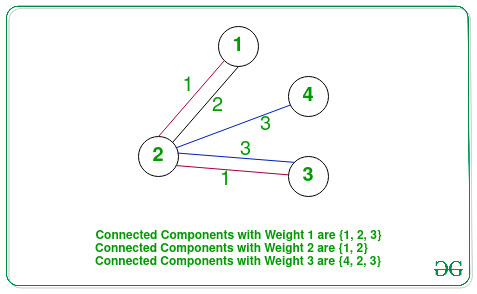Related Articles

# Maximum product of a pair of nodes from largest connected component in a Graph

• Difficulty Level : Hard
• Last Updated : 05 Jul, 2021

Given an undirected weighted graph G consisting of N vertices and M edges, and two arrays Edges[] and Weight[] consisting of M edges of the graph and weights of each edge respectively, the task is to find the maximum product of any two vertices of the largest connected component of the graph, formed by connecting all edges with the same weight.

Examples:

Input: N = 4, Edges[][] = {{1, 2}, {1, 2}, {2, 3}, {2, 3}, {2, 4}}, Weight[] = {1, 2, 1, 3, 3}
Output: 12
Explanation:• Components of edges of weight 1, 1 ↔ 2 ↔ 3. The maximum product of any two vertices of this component is 6.
• Components of edges of weight 2, 1 ↔ 2. The maximum product of any two vertices of this component is 2.
• Components of edges of weight 3, 4 ↔ 2 ↔ 3. The maximum product of any two vertices of this component is 12.

Therefore, the maximum product among all the connected components of size 3 (which is maximum) is 12.

Input: N = 5, Edges[][] = {{1, 5}, {2, 5}, {3, 5}, {4, 5}, {1, 2}, {2, 3}, {3, 4}}, Weight[] = {1, 1, 1, 1, 2, 2, 2}
Output: 20

Approach: The given problem can be solved by performing the DFS traversal on the given graph and maximize the product of the first and second maximum node for all the connected components of the same weight. Follow the steps below to solve the problem:

• Store all the edges corresponding to all the unique weight in a map M.
• Initialize a variable, say res as 0 to store the maximum product of any two nodes of the connected components of the same weights.
• Traverse the map and for each key as weight create a graph by connecting all the edges mapped with the particular weight and perform the following operations:
• Find the value of the maximum(say M1) and the second maximum(say M2) node’s value and the size of all the connected components of the graph by performing the DFS Traversal on the created graph.
• Update the value of res to the maximum of res, M1, and M2 if the size of the currently connected components is at least the largest size connected component found previously.
• After completing the above steps, print the value of res as the maximum product.

Below is the implementation of the above approach:

## C++

 `// C++ program for the above approach` `#include ``using` `namespace` `std;` `// Stores the first and second largest``// element in a connected component``int` `Max, sMax;` `// Stores the count of nodes``// in the connected components``int` `cnt = 0;` `// Function to perform DFS Traversal``// on a given graph and find the first``// and the second largest elements``void` `dfs(``int` `u, ``int` `N, vector<``bool``>& vis,``         ``vector >& adj)``{``    ``// Update the maximum value``    ``if` `(u > Max) {``        ``sMax = Max;``        ``Max = u;``    ``}` `    ``// Update the second max value``    ``else` `if` `(u > sMax) {``        ``sMax = u;``    ``}` `    ``// Increment size of component``    ``cnt++;` `    ``// Mark current node visited``    ``vis[u] = ``true``;` `    ``// Traverse the adjacent nodes``    ``for` `(``auto` `to : adj[u]) {` `        ``// If to is not already visited``        ``if` `(!vis[to]) {``            ``dfs(to, N, vis, adj);``        ``}``    ``}` `    ``return``;``}` `// Function to find the maximum``// product of a connected component``int` `MaximumProduct(``    ``int` `N, vector > Edge,``    ``vector<``int``> wt)``{``    ``// Stores the count of edges``    ``int` `M = wt.size();` `    ``// Stores all the edges mapped``    ``// with a particular weight``    ``unordered_map<``int``,``                  ``vector > >``        ``mp;` `    ``// Update the map mp``    ``for` `(``int` `i = 0; i < M; i++)``        ``mp[wt[i]].push_back(Edge[i]);` `    ``// Stores the result``    ``int` `res = 0;` `    ``// Traverse the map mp``    ``for` `(``auto` `i : mp) {` `        ``// Stores the adjacency list``        ``vector > adj(N + 1);` `        ``// Stores the edges of``        ``// a particular weight``        ``vector > v = i.second;` `        ``// Traverse the vector v``        ``for` `(``int` `j = 0; j < v.size(); j++) {` `            ``int` `U = v[j].first;``            ``int` `V = v[j].second;` `            ``// Add an edge``            ``adj[U].push_back(V);``            ``adj[V].push_back(U);``        ``}` `        ``// Stores if a vertex``        ``// is visited or not``        ``vector<``bool``> vis(N + 1, 0);` `        ``// Stores the maximum``        ``// size of a component``        ``int` `cntMax = 0;` `        ``// Iterate over the range [1, N]``        ``for` `(``int` `u = 1; u <= N; u++) {` `            ``// Assign Max, sMax, count = 0``            ``Max = sMax = cnt = 0;` `            ``// If vertex u is not visited``            ``if` `(!vis[u]) {` `                ``dfs(u, N, vis, adj);` `                ``// If cnt is greater``                ``// than cntMax``                ``if` `(cnt > cntMax) {` `                    ``// Update the res``                    ``res = Max * sMax;``                    ``cntMax = cnt;``                ``}` `                ``// If already largest``                ``// connected component``                ``else` `if` `(cnt == cntMax) {` `                    ``// Update res``                    ``res = max(res, Max * sMax);``                ``}``            ``}``        ``}``    ``}` `    ``// Return res``    ``return` `res;``}` `// Driver Code``int` `main()``{``    ``int` `N = 5;``    ``vector > Edges``        ``= { { 1, 2 }, { 2, 5 }, { 3, 5 }, { 4, 5 }, { 1, 2 }, { 2, 3 }, { 3, 4 } };` `    ``vector<``int``> Weight = { 1, 1, 1, 1,``                           ``2, 2, 2 };``    ``cout << MaximumProduct(N, Edges, Weight);` `    ``return` `0;``}`
Output:
`20`

Time Complexity: O(N2 * log N + M)
Auxiliary Space: O(N2)

Attention reader! Don’t stop learning now. Get hold of all the important DSA concepts with the DSA Self Paced Course at a student-friendly price and become industry ready.  To complete your preparation from learning a language to DS Algo and many more,  please refer Complete Interview Preparation Course.

In case you wish to attend live classes with experts, please refer DSA Live Classes for Working Professionals and Competitive Programming Live for Students.

My Personal Notes arrow_drop_up